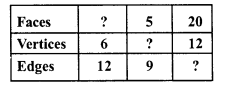## RD Sharma Class 8 Solutions Chapter 19 Visualising Shapes Ex 19.1

These Solutions are part of RD Sharma Class 8 Solutions. Here we have given RD Sharma Class 8 Solutions Chapter 19 Visualising Shapes Ex 19.1

Other Exercises

Question 1.
What is the least number of planes that can enclose a solid ? What is the name of the solid ?
Solution:
The least number of planes that can enclose a solid is called a Tetrahedron.

Question 2.
Can a polyhedron have for its faces :
(i) three triangles ?
(ii) four triangles ?
(iii) a square and four triangles ?
Solution:
(i) No, polyhedron has three faces.
(ii) Yes, tetrahedron has four triangles as its faces.
(iii) Yes, a square pyramid has a square as its base and four triangles as its faces.

Question 3.
Is it possible to have a polyhedron with any given number of faces ?
Solution:
Yes, it is possible if the number of faces is 4 or more.

Question 4.
Is a square prism same as a cube ?
Solution:
Yes, a square prism is a cube.

Question 5.
Can a polyhedron have 10 faces, 20 edges and 15 vertices ?
Solution:
No, it is not possible as By Euler’s formula
F + V = E + 2
⇒ 10 + 15 = 20 + 2
⇒ 25 = 22
Which is not possible

Question 6.
Verify Euler’s formula for each of the following polyhedrons :Solution:
(i) In this polyhedron,
Number of faces (F) = 7
Number of edges (E) = 15
Number of vertices (V) = 10
According to Euler’s formula,
F + V = E + 2
⇒ 7 + 10 = 15 + 2
⇒ 17 = 17
Which is true.
(ii) In this polyhedron,
Number of faces (F) = 9
Number of edges (E) = 16
Number of vertices (V) = 9
According to Euler’s formula,
F + V = E + 2
⇒ 9 + 9 = 16 + 2
⇒ 18 = 18
Which is true.
(iii) In this polyhedron,
Number of faces (F) = 9
Number of edges (E) =18
Number of vertices (V) = 11
According to Euler’s formula,
F + V = E + 2
⇒ 9 + 11 = 18 + 2
⇒ 20 = 20
Which is true.
(iv) In this polyhedron,
Number of faces (F) = 5
Number of edges (E) = 8
Number of vertices (V) = 5
According to Euler’s formula,
F + V = E + 2
⇒ 5 + 5 = 8 + 2
⇒ 10 = 10
Which is true.
(v) In the given polyhedron,
Number of faces (F) = 9
Number of edges (E) = 16
Number of vertices (V) = 9
According to Euler’s formula,
F + V = E + 2
⇒ 9 + 9 = 16 + 2
⇒ 18 = 18
Which is true.

Question 7.
Using Euler’s formula, find the unknown:Solution:
We know that Euler’s formula is
F + V = E + 2
(i) F + 6 = 12 + 2
⇒ F + 6 = 14
⇒ F = 14 – 6 = 8
Faces = 8
(ii) F + V = E + 2
⇒ 5 + V = 9 + 2
⇒ 5 + V = 11
⇒ V = 11 – 5 = 6
Vertices = 6
(iii) F + V = E + 2
⇒ 20 + 12 = E + 2
⇒ 32 = E + 2
⇒ E = 32 – 2 = 30
Edges = 30

Hope given RD Sharma Class 8 Solutions Chapter 19 Visualising Shapes Ex 19.1 are helpful to complete your math homework.

If you have any doubts, please comment below. Learn Insta try to provide online math tutoring for you.